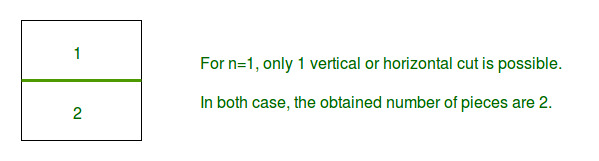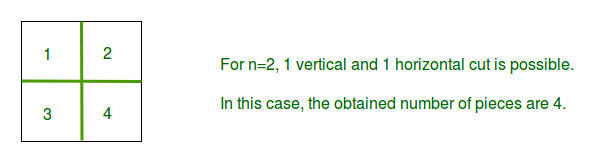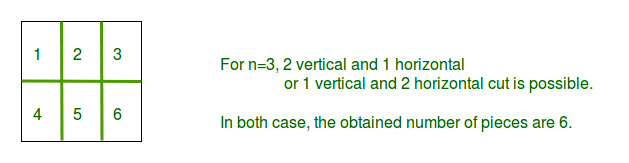# Maximum number of pieces in N cuts

Given a square piece and a total number of cuts available n, Find out the maximum number of rectangular or square pieces of equal size that can be obtained with n cuts. The allowed cuts are horizontal and vertical cut.

Note: Stacking and folding is not allowed.

Examples:

```Input : n = 1
Output : 2
Explanation :Input : n = 2
Output : 4
Explanation :Input : n = 3
Output : 6
Explanation :```

## Recommended: Please try your approach on {IDE} first, before moving on to the solution.

Given is n which is the number of allowed cuts. As it is required to maximize number of pieces after n cuts, So number of horizontal cuts will be equal to number of vertical cuts. This can be prove using differentiation. So number of horizontal cut will be n/2. and vertical cuts will be n-n/2.

So number of pieces = (horizontal cut + 1) * (vertical cut + 1).

Program:

## C++

 `// C++ program to find maximum no of pieces ` `// by given number of cuts ` `#include ` `using` `namespace` `std; ` ` `  `// Function for finding maximum pieces ` `// with n cuts. ` `int` `findMaximumPieces(``int` `n) ` `{ ` `    ``// to maximize number of pieces ` `    ``// x is the horizontal cuts ` `    ``int` `x = n / 2; ` ` `  `    ``// Now (x) is the horizontal cuts ` `    ``// and (n-x) is vertical cuts, then ` `    ``// maximum number of pieces = (x+1)*(n-x+1) ` `    ``return` `((x + 1) * (n - x + 1)); ` `} ` ` `  `// Driver code ` `int` `main() ` `{ ` ` `  `    ``// Taking the maximum number of cuts allowed as 3 ` `    ``int` `n = 3; ` ` `  `    ``// Finding and printing the max number of pieces ` `    ``cout << ``"Max number of pieces for n = "` `<< n ` `         ``<< ``" is "` `<< findMaximumPieces(3); ` ` `  `    ``return` `0; ` `} `

## Java

 `// Java program to find maximum  ` `// no of pieces by given number ` `// of cuts  ` `import` `java.util.*; ` ` `  `class` `GFG ` `{ ` `// Function for finding maximum ` `// pieces with n cuts.  ` `public` `static` `int` `findMaximumPieces(``int` `n)  ` `{  ` `    ``// to maximize number of pieces  ` `    ``// x is the horizontal cuts  ` `    ``int` `x = n / ``2``;  ` ` `  `    ``// Now (x) is the horizontal cuts  ` `    ``// and (n-x) is vertical cuts, then  ` `    ``// maximum number of pieces = (x+1)*(n-x+1)  ` `    ``return` `((x + ``1``) * (n - x + ``1``));  ` `}  ` ` `  `// Driver code ` `public` `static` `void` `main (String[] args)  ` `{ ` `    ``// Taking the maximum number  ` `    ``// of cuts allowed as 3  ` `    ``int` `n = ``3``;  ` `     `  `    ``// Finding and printing the  ` `    ``// max number of pieces  ` `    ``System.out.print(``"Max number of pieces for n = "` `+ ` `                   ``n + ``" is "` `+ findMaximumPieces(``3``));  ` `         `  `} ` `} ` ` `  `// This code is contributed by Kirti_Mangal `

## Python 3

 `# Python 3 program to find maximum no of pieces ` `# by given number of cuts ` `  `  `# Function for finding maximum pieces ` `# with n cuts. ` `def` `findMaximumPieces(n): ` ` `  `    ``# to maximize number of pieces ` `    ``# x is the horizontal cuts ` `    ``x ``=` `n ``/``/` `2` `  `  `    ``# Now (x) is the horizontal cuts ` `    ``# and (n-x) is vertical cuts, then ` `    ``# maximum number of pieces = (x+1)*(n-x+1) ` `    ``return` `((x ``+` `1``) ``*` `(n ``-` `x ``+` `1``)) ` `  `  `# Driver code ` `if` `__name__ ``=``=` `"__main__"``: ` `  `  `    ``#Taking the maximum number of cuts allowed as 3 ` `    ``n ``=` `3` `  `  `    ``# Finding and printing the max number of pieces ` `    ``print``(``"Max number of pieces for n = "` `+``str``( n) ` `         ``+``" is "` `+` `str``(findMaximumPieces(``3``))) ` ` `  `# This code is contributed by ChitraNayal `

## C#

 `// C# program to find maximum  ` `// no of pieces by given number ` `// of cuts  ` `using` `System; ` ` `  `class` `GFG ` `{ ` ` `  `// Function for finding maximum ` `// pieces with n cuts.  ` `public` `static` `int` `findMaximumPieces(``int` `n)  ` `{  ` `    ``// to maximize number of pieces  ` `    ``// x is the horizontal cuts  ` `    ``int` `x = n / 2;  ` ` `  `    ``// Now (x) is the horizontal   ` `    ``// cuts and (n-x) is vertical  ` `    ``// cuts, then maximum number  ` `    ``// of pieces = (x+1)*(n-x+1)  ` `    ``return` `((x + 1) * (n - x + 1));  ` `}  ` ` `  `// Driver code ` `static` `public` `void` `Main () ` `{ ` `    ``// Taking the maximum number  ` `    ``// of cuts allowed as 3  ` `    ``int` `n = 3;  ` `     `  `    ``// Finding and printing the  ` `    ``// max number of pieces  ` `    ``Console.Write(``"Max number of pieces for n = "` `+ ` `                ``n + ``" is "` `+ findMaximumPieces(3));  ` `} ` `} ` ` `  `// This code is contributed by Mahadev `

## PHP

 ` `

Output:

```Max number of pieces for n = 3 is 6
```

Attention reader! Don’t stop learning now. Get hold of all the important DSA concepts with the DSA Self Paced Course at a student-friendly price and become industry ready.

My Personal Notes arrow_drop_upCheck out this Author's contributed articles.

If you like GeeksforGeeks and would like to contribute, you can also write an article using contribute.geeksforgeeks.org or mail your article to contribute@geeksforgeeks.org. See your article appearing on the GeeksforGeeks main page and help other Geeks.

Please Improve this article if you find anything incorrect by clicking on the "Improve Article" button below.

Article Tags :
Practice Tags :

2

Please write to us at contribute@geeksforgeeks.org to report any issue with the above content.